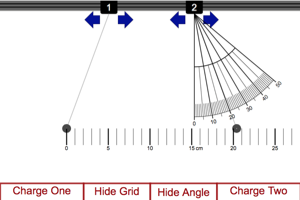Programs for Force Electric
Below are all the programs that might be used with this topic. Click on "See Resources" to see if there are any student directions, lab sheets or other materials that have been created for this program.
Coulomb's Law Lab

LabForce Electric Mini Lab

LabForce Electric MiniLab with Numbers

LabForce Electric in a Line Problem

HomeworkForce Electric Triangular Arrangement Problem

HomeworkForce Electric in a Box Problem

HomeworkForce Electric Between Two Charges

HomeworkCharge Deflected by Capacitor

HomeworkElectric Field Line

Homework